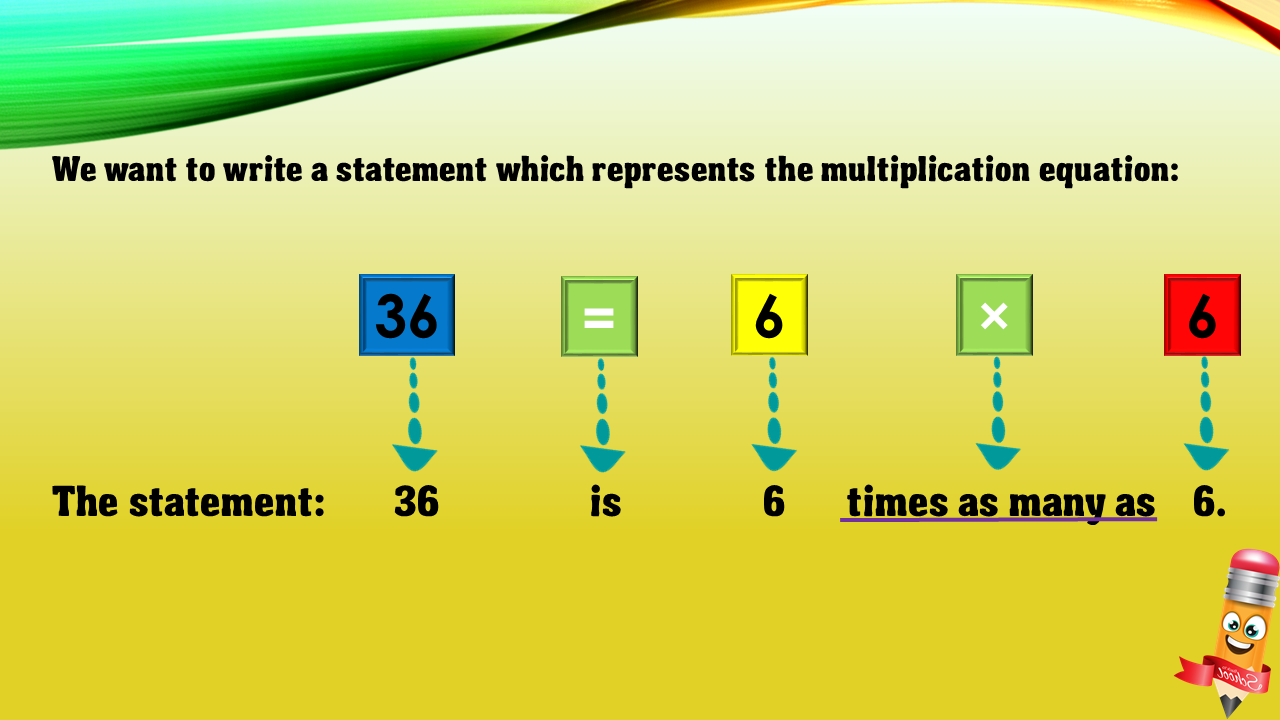1
visibility

Choose one statement which represents the equation: 36 = 6×6 ?

• A

36 is 6 more than 6.

• B

6 is 6 less than 36.

• C

36 is 6 times as many as 6.

• D

36 times as many as 6 is 6.